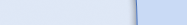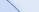Шутки

### Why you should learn to use algebra . . .

Why you should learn to use algebra . . .

After applying some simple algebra to some trite phrases and cliches a new understanding can be reached of the secret to wealth and success. Here it goes.

Knowledge is Power Time is Money and as every engineer knows, Power is Work over Time.

So, substituting algebraic equations for these time worn bits of wisdom, we get:

K = P (1) T = M (2) P = W/T (3)

Now, do a few simple substitutions:

Put W/T in for P in equation (1), which yields: K = W/T (4)

Put M in for T into equation (4), which yields:

K = W/M (5).

Now we've got something. Expanding back into English, we get:

Knowledge equals Work over Money.

What this MEANS is that:

1. The More You Know, the More Work You Do, and 2. The More You Know, the Less Money You Make.

Solving for Money, we get:

M = W/K (6) Money equals Work Over Knowledge.

From equation (6) we see that Money approaches infinity as Knowledge approaches 0, regardless of the Work done.

What THIS MEANS is:

The More you Make, the Less you Know.

Solving for Work, we get

W = M K (7) Work equals Money times Knowledge

From equation (7) we see that Work approaches 0 as Knowledge approaches 0.

What THIS MEANS is:

The stupid rich do little or no work.

Working out the socioeconomic implications of this breakthrough is left as an exercise for the reader.

#### Категория: Science (Наука); At Work (Работа)

Просмотров материала: 1090

Читайте также из категории Science (Наука):

ТОП-5 категории Science (Наука):